# Pallet Toolbar

#### StarUML

 Pallet Toolbar

The Pallet toolbar contains the following functions for selecting and creating elements in the diagram.

Common Pallet Tool

The following functions are always available in the Pallet toolbar regardless of the diagram types.

 Tool Description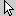Select The most basic tool that selects, moves or resizes an element in the diagram.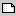Note Creates a note element in the current diagram.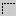Note Link Links a note in the current diagram to another element.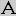Text Creates a string element in the current diagram.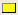Rectangle Create a figure of  rectangle in the current diagram.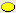Ellipse Creates a figure of  ellipse in the current diagram.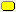Rounded Rectangle Creates a figure of  rounded rectangle in the current diagram.

Pallet Tool by Diagram Types

The following functions create elements by diagram types.

 Tool Description DiagramSelect The most basic tool that selects, moves or resizes an element in the diagram. All diagrams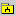Subsystem Creates a subsystem element in the current diagram. Class Diagram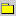Package Creates a package element in the current diagram.. Class Diagram, Component Diagram, Deployment Diagram, UseCase Diagram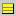Class Creates a class element in the current diagram. Class Diagram, Composite Diagram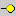Interface Creates an interface element in the current diagram. Class Diagram, Component Diagram, Composite Diagram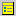Enumeration Creates an enumeration element in the current diagram. Class Diagram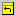Signal Creates a signal element in the current diagram. Class Diagram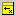Except Creates an exception element in the current diagram. Class Diagram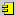Component Creates a component element in the current diagram. Component Diagram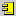ComponentInstance Creates a component instance element in the current diagram. Component Diagram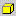Node Creates a node element in the current diagram. Deployment Diagram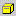NodeInstance Creates a node instance element in the current diagram. Component Diagram, Deployment Diagram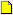Artifact Creates a artifact in the current diagram. UseCase Diagram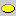UseCase Creates a usecase element in the current diagram. UseCase Diagram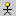Actor Creates an actor element in the current diagram. UseCase Diagram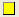SystemBoundary Creates an system boundary in the current diagram. UseCase Diagram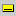Object Creates an object element in the current diagram. Class Diagram, Sequence Diagram, Collaboration Diagram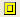Part Creates a  Part element with a Classifier in the current diagram. Class Diagram, Component Diagram, Deployment Diagram, Composite Diagram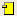Port Creates a  Port element with a Classifier in the current diagram. Class Diagram, Component Diagram, Deployment Diagram, Composite Diagram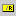ClassifierRole Creates a ClassifierRole element in the current diagram. Sequence Role Diagram, Collaboration Role Diagram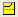Combined Fragment Creates a Combined Fragment element in the current diagram. Sequence Diagram, Sequence Role Diagram, Collaboration Diagram, Collaboration Role Diagram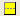Interaction Operand Creates a  Interaction Operand element with a Combined Fragment in the current diagram. Sequence Diagram, Sequence Role Diagram, Collaboration Diagram, Collaboration Role Diagram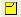Frame Creates a Frame element in the current diagram Sequence Diagram, Sequence Role Diagram, Collaboration Diagram, Collaboration Role Diagram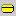CompositeState Creates a CompositeState element in the current diagram. Statechart Diagram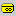SubmachineState Creates a SubmachineState element in the current diagram. Statechart Diagram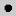InitialState Creates an InitialState (Pseudostate) element in the current diagram. Statechart Diagram, Activity Diagram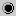FinalState Creates a FinalState element in the current diagram. Statechart Diagram, Activity Diagram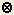Flow Final Creates a DeepHistory(FlowFinalState) element in the current diagram. Statechart Diagram, Activity Diagram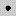ChoicePoint Creates a Choice (Pseudostate) element in the current diagram. Statechart Diagram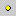JunctionPoint Creates a Junction (Pseudostate) element in the current diagram. Statechart Diagram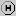ShallowHistory Creates a ShallowHistory (Pseudostate) element in the current diagram. Statechart Diagram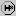DeepHistory Creates a DeepHistory (Pseudostate) element in the current diagram. Statechart Diagram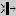Synchronization Creates a Synchronization (Pseudostate) element in the current diagram. Statechart Diagram, Activity Diagram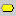ActionState Creates an ActionState element in the current diagram. Activity Diagram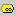SubactivityState Creates a Subactivity State element in the current diagram. Activity Diagram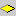Decision Creates a Decision (Pseudostate) element in the current diagram. Activity Diagram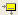ObjectFlow Creates a ObjectFlowState element in the current diagram. Activity Diagram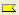Signal Accept State Creates a SignalAcceptState element in the current diagram. Activity Diagram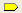Signal Send State Creates a SignalSendState element in the current diagram. Activity Diagram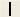Swimlane(Vertical) Creates a Swimlane by vertical solid lines in the current diagram. Activity Diagram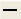Swimlane(Horizontal) Creates a Swimlane by horizontal solid lines in the current diagram. Activity Diagram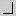Association Links a semantic association between two classes in the current diagram. Class Diagram, Component Diagram, Deployment Diagram, UseCase Diagram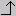DirectedAssociation Links a semantic association between two classes in the current diagram. Class Diagram, Deployment Diagram, UseCase Diagram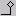Aggregation Links a semantic association between two classes in the current diagram. Class Diagram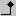Composition Links a semantic association between two classes in the current diagram. Class Diagram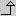Generalization Links a generalized element and a specialized element with a generalization relation in the current diagram. Class Diagram, UseCase DiagramDependency Links two elements with a dependency relation in the current diagram. Class Diagram, Component Diagram, Deployment Diagram, UseCase Diagram, Composite Diagram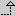Realization Links a specification element and its implementation element with a realization relation in the current diagram. Class Diagram, Component Diagram, Composite Diagram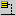AssociationClass Links a class and an association in the current diagram so that the association itself can have the significance of a class. Class Diagram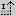Include Links two UseCases with an Include relation in the current diagram so that one UseCase includes the other UseCase behaviors. UseCase Diagram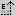Extend Links two UseCases with an Extend relation in the current diagram so that one UseCase can be extended with the behaviors defined in the other UseCase. UseCase Diagram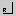AssociationRole Links two roles with an AssociationRole in the current diagram. Collaboration Role Diagram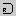SelfAssociationRole Creates an AssociationRole from one role to the same role in the current diagram. Collaboration Role Diagram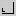Link Links two objects in the current diagram. Class Diagram, Collaboration Diagram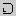SelfLink Links an object with itself in the current diagram. Class Diagram, Collaboration Diagram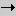ForwardMessage Defines a message between two roles in the current diagram. Sequence Role Diagram, Collaboration Role Diagram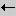ReverseMessage Defines a message between two roles in the current diagram. Sequence Role Diagram, Collaboration Role Diagram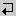SelfMessage Creates a message from a role to the same role in the current diagram. Sequence Role Diagram, Collaboration Role DiagramForwardStimulus Defines a stimulus between two objects in the current diagram. Sequence Diagram, Collaboration DiagramReverseStimulus Defines a stimulus between two objects in the current diagram. Sequence Diagram, Collaboration DiagramSelfStimulus Creates a stimulus from an object to the same object in the current diagram. Sequence Diagram, Collaboration Diagram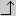Transition Links a source state and a target state with a transition in the current diagram. Statechart Diagram, Activity Diagram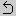SelfTransition Links an original state and a target state with a transition in the current diagram. Statechart Diagram, Activity Diagram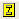Connector Links a original feature and a target feature with a connector in the current diagram. Class Diagram, Component Diagram, Deployment Diagram, Composite Diagram